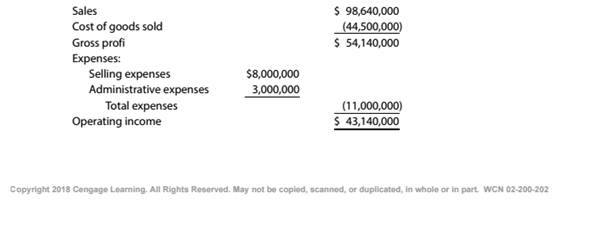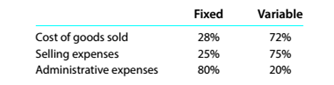Chapter 11, Problem 11.2.1P

Chapter
Section
Textbook Problem

Break-even sales under present and proposed conditions Kearney Company, operating at full capacity, sold 400,000 units at a price of \$246.60 per unit during 20Y5. Its income statement for 20Y5 is as follows:The division of costs between fixed and variable is as follows:Management is considering a plant expansion program that will permit an increase of \$8,631,000 (35.000 units at \$246.60) in yearly sales. The expansion will increase fixed costs by \$3,600,000 but will not affect the relationship between sales and variable costs.InstructionsDetermine for 20Y5 the total fixed costs and the total variable costs.

To determine

Concept Introduction:

Cost Volume Profit (CVP) Analysis:

The Cost Volume Profit analysis is the analysis of the relation between cost, volume, and profit of a product. It analyzes the cost and profits at the different level of production, in order to determine the breakeven point and required the level of sales to earn the desired profit.

Contribution margin means the margin that is left with the company after recovering variable cost out of revenue earned by selling smart phones. The formula:

Contribution margin = Sales - Variable cost.

Similarly contribution margin ratio = Contribution/sales

To Calculate:

The total fixed costs and total variable costs

Explanation

The total fixed costs and total variable costs are calculated as follows:

 Fixed Variable Cost of Goods Sold \$ 12,460,000 \$ 32,040,000 (44500000*28%) (44500000*72%) Selling Expenses \$ 2,000,00...

Still sussing out bartleby?

Check out a sample textbook solution.

See a sample solution

The Solution to Your Study Problems

Bartleby provides explanations to thousands of textbook problems written by our experts, many with advanced degrees!

Get Started

Find more solutions based on key concepts